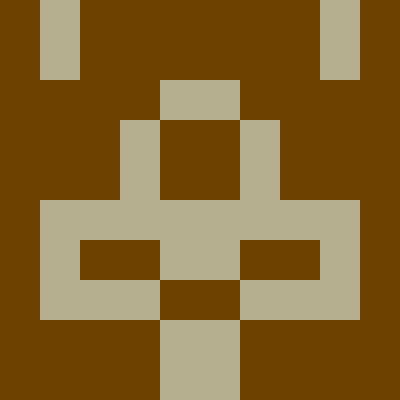Copied to clipboard

#### Flag this post as spam?

This post will be reported to the moderators as potential spam to be looked at

• Bobi 271 posts 724 karma points
Mar 31, 2017 @ 07:590

## Counting Results

Anyone know how to find total results when searching blog posts in Articulate?

I want to display on the first page:

Your search for "text" matched 35 results.

Showing results 1 to 20. ... [results] [pager]

Next Page: Your search for "text" matched 35 results.

Showing results 21 to 35. ... [results] [pager]

I'm trying to find the number of total results (in the example here it's 35).

I am using the following logic, but it's not giving me the total results because @Model.Children.Count() only gives the count for the results on the page, not the total results.

``````var totalPages = (int)Math.Ceiling((decimal)Model.Children.Count() / Model.PageSize);
var currentPage = Math.Max(1, Math.Min(totalPages, Model.Pages.CurrentPageIndex));

var startRecord = ((currentPage - 1) * Model.Pages.PageSize) + 1;
var endRecord = Math.Min(Model.Children.Count(), (startRecord - 1) + Model.Pages.PageSize);
``````

I need to replace Model.Children.Count() with the total number of results (totalResults, if you will).

Any ideas?